#### Machine learning in R for credit scoring

The problem targeted at this Machine Learning exercise is to predict
creditworthiness of a client at a German Bank, based on 20 quantitative
and qualitative characteristics of a client.

Based on historical records, clients fall into two categories:

• 'good', i.e. credit-worthy clients, who would most probably repay credit.
• 'bad', most probably would not repay.

The cost of the 'wrong' decision, i.e. giving credit to a client who would not
return it, is higher. This cost should be incorporated via 'cost matrix', specifying
that such a mistake is 5 times costlier (can be any number higher than 1).

The data can be found at UCI Machine Learning)
repository.

## Motivation for exploring the problem in R

This machine learning exercise is going to be coded in Python, as Python's `scikit-learn`,
in general, is more powerful in model tuning than R. However, prior to doing that,
an attempt is made to quickly explore the data set in R for the following reasons:

• to get acquainted with salient characteristics of the data and visualize data
• develop a feeling what accuracy could be for a range of simple algorithms in R
• try different coding schemes for class variables, namely:
• grouping them together
• coding them separately as dummy variables
• explore what effect penalty cost-matrix can have on results
• explore directions for further model tuning:
• is collinearity present among features?
• are there non-informative features in the data set?
• what kind of feature engineering could be attempted?

## Load data into R

First we need to load data into R and assign names to features, as the data set
does not have header.

Note, that category codings ('A5', 'A13' etc) are appended
to numerical features, whereas categorical features are appended with “_”. The latter will
ease reading names of the features when dummies are expanded and dummy labels ('A101', 'A72' etc)
are appended after “_”. The meaning of 'A**' labels can be found at this page).

As example:

• `num_duration_A2` means 'duration in month'
• `chk_account_A12` would mean application between 0 and DM200.
``````source('./functions/plotImp.R')    # for plotting variable importance
library(SIT)                       # for `spl` function

# enter feature names
.names <- spl("chk_account_,num_duration_A2,history_,purpose_,num_amount_A5,svn_account_,empl_status_,num_income_share_A8,marital_status_,guarantor_,num_residence_A11,property_,num_age_A13,other_loans_,housing_,num_credits_A16,job_,num_dependants_A18,telephone_,foreign_,status")

# make vector of .colClasses
.num         <- grepl('^num_', .names)  # search for numeric data
.colClasses  <- ifelse(.num, "numeric", "factor")

# read data into R
col.names = .names,
colClasses = .colClasses)
``````

## Explore data

Let's start our data exploration with assigning outcome labels 'good'/'bad' to
½ coded status variable and checking composition of our data set.

``````levels(raw\$status) <- c('good', 'bad')
summary(raw\$status)
``````
```## good  bad
##  700  300
```

There are two conclusions here:

• the data set is slightly unbalanced
• the base accuracy, that is always assigning majority class to outcome event, is 0.7.
In banking parlor base accuracy is the probability of always advancing a credit,
irregardless of client's profile. This is the figure we will target to improve upon.

Both application duration and the amount of money asked for are right-skewed.

``````par(mfrow=c(1,2))
hist(raw\$num_duration_A2, main='Duration distribution', xlab='months')
hist(raw\$num_amount_A5, main='Amount distribution', xlab = 'DM')
``````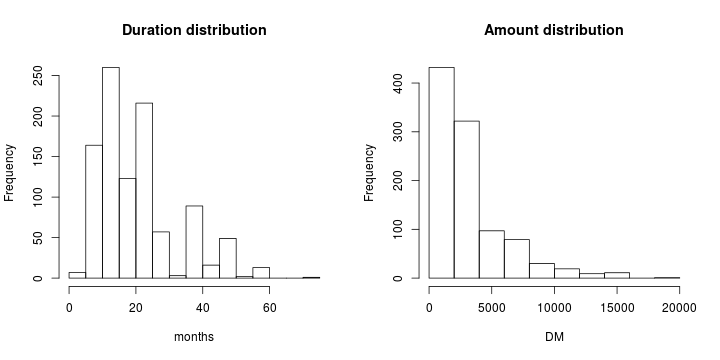Searching for what constitutes the bulk of the applications:

``````quantile(raw\$num_amount_A5, .8)
``````
```##  80%
## 4720
```
``````library(dplyr)
rawSorted <- filter(raw, num_amount_A5 > quantile(num_amount_A5,.8))
median(rawSorted\$num_duration_A2)
``````
```##  36
```

we will conclude that 80% of the applications are for the sums under DM 5'000 and
duration below 36 months.

## Split train/test

To train hypeparameters of ML algos and to check generalization ability of our models
we need to split `raw` data set into train/test subsets.

NOTE:

There are two sets showing two possible representations of the same features:

• `xTrain`, the set where categorical features are expanded explicitly and coded as 0/1 dummies.
• `xTrainG`, the set where categorical features are grouped (kept together as character strings).
This possibility is due to specific treatment of
grouped categorical variables by trees when they are provided as x/y separately,
as opposed to formula representation (see Max Kuhn, 'Applied Predictive Modeling', Ch.14,
for extended discussion)
``````# dummies expanded explicitly
.x <- model.matrix(~.-1, data=raw[,-21])
.y <- raw[,21]

library(caTools)
set.seed(1)
ind   <- sample.split(raw\$status, SplitRatio = .7)

xTrain <- .x[ ind,]
xTest  <- .x[!ind,]

yTrain <- .y[ ind]
yTest  <- .y[!ind]

# grouped categorical variables for trees methods RF, C5.0 etc.
xTrainG <- raw[ ind,-21]
xTestG  <- raw[!ind,-21]
``````

## Building models

Below I will build several ensemble models, including Random Forest, boosted trees, and C5.0, providing rational for using a particular method. All
the models will be built on both grouped and separate feature data sets comparing
model performance side by side. All of these are non-linear, highly-performant black boxes,
that provide most accurate predictions while giving little insight into what should
drive a credit officer's decision making process.

I will conclude with a regularized logistic regression, less accurate, but
more interpretable.

### Random Forest

Decision trees are powerful and popular tools, but they suffer
from high variability: if one samples a slightly different
data set, splitting rules may change completely. That was overcome by bagging
(bootstrap averaging) many trees at once and averaging
the outcome in a black box called Random Forest.

In humane language, one grabs a random portion of the data – random both on
features and cases – and builds on it a fully grown decision tree. This randomization
allows capturing important nuances of the data. After a number of different
trees have been trained, they are combined into a single black box where outcomes are
decided:

• either by averaging predicted probabilities from different tress
• or by majority votes.

NOTE:

Random Forest is an example of bagged trees. In general, bagging, as a statistical
learning technique, can be applied
to any 'weak' learner, e.g. Naïve Bayes or k-Nearest Neighbour classifyer.

Below is an example of tuning Random Forest from `randomForest` package via
`caret` function `train`.

Training model (tuning hyperparameters via cross-validation) is a time-consuming
process. This is why I turn on multi-threading and run R process on 2 cores:

``````library(doMC)
registerDoMC(2)     # 2 cores
library(caret)
``````

Then, let's build a train control, which will be used across all the models for consistency,
tune grid for RF, and train the model.

``````ctrl   <- trainControl(method = 'repeatedcv', # 10-fold 5-repeats cv
number=10,
repeats=5,
allowParallel = T)        # parallelize execution

gridRF   <- expand.grid(mtry=seq(5,30,by=2))     # training grid, # of features to split upon

set.seed(1)         # to ensure consistency in sampling across all models
mod_rf <- train(x = xTrain,
y = yTrain,
method    ='rf',
tuneGrid  = gridRF,
trControl = ctrl)

plot(mod_rf, main='Tuning RF on separate dummies')
``````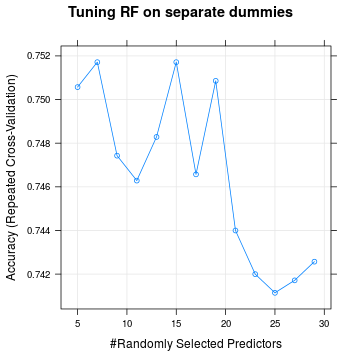It appears that best result is achieved at 7 splits.

Before I will proceed further it's worth noting that there is no need to memorize
the list of all possible models and tuning parameters for a particular
model.

``````names(getModelInfo())
``````
```##    "ada"                 "AdaBag"              "AdaBoost.M1"
##    "amdai"               "ANFIS"               "avNNet"
##    "bag"                 "bagEarth"            "bagEarthGCV"
##   "bagFDA"              "bagFDAGCV"           "bayesglm"
##   "bdk"                 "binda"               "blackboost"
##   "Boruta"              "brnn"                "bstLs"
##   "bstSm"               "bstTree"             "C5.0"
##   "C5.0Cost"            "C5.0Rules"           "C5.0Tree"
##   "cforest"             "chaid"               "CSimca"
##   "ctree"               "ctree2"              "cubist"
##   "DENFIS"              "dnn"                 "earth"
##   "elm"                 "enet"                "enpls.fs"
##   "enpls"               "evtree"              "extraTrees"
##   "fda"                 "FH.GBML"             "FIR.DM"
##   "foba"                "FRBCS.CHI"           "FRBCS.W"
##   "FS.HGD"              "gam"                 "gamboost"
##   "gamLoess"            "gamSpline"           "gaussprLinear"
##   "gaussprPoly"         "gaussprRadial"       "gbm"
##   "gcvEarth"            "GFS.FR.MOGAL"        "GFS.GCCL"
##   "GFS.LT.RS"           "GFS.THRIFT"          "glm"
##   "glmboost"            "glmnet"              "glmStepAIC"
##   "gpls"                "hda"                 "hdda"
##   "HYFIS"               "icr"                 "J48"
##   "JRip"                "kernelpls"           "kknn"
##   "knn"                 "krlsPoly"            "krlsRadial"
##   "lars"                "lars2"               "lasso"
##   "lda"                 "lda2"                "leapBackward"
##   "leapForward"         "leapSeq"             "Linda"
##   "lm"                  "lmStepAIC"           "LMT"
##   "logicBag"            "LogitBoost"          "logreg"
##   "lssvmLinear"         "lssvmPoly"           "lssvmRadial"
##   "lvq"                 "M5"                  "M5Rules"
##   "mda"                 "Mlda"                "mlp"
##  "mlpWeightDecay"      "multinom"            "nb"
##  "neuralnet"           "nnet"                "nodeHarvest"
##  "oblique.tree"        "OneR"                "ORFlog"
##  "ORFpls"              "ORFridge"            "ORFsvm"
##  "pam"                 "parRF"               "PART"
##  "partDSA"             "pcaNNet"             "pcr"
##  "pda"                 "pda2"                "penalized"
##  "PenalizedLDA"        "plr"                 "pls"
##  "plsRglm"             "polr"                "ppr"
##  "protoclass"          "qda"                 "QdaCov"
##  "qrf"                 "qrnn"                "rbf"
##  "rbfDDA"              "rda"                 "relaxo"
##  "rf"                  "rFerns"              "RFlda"
##  "ridge"               "rknn"                "rknnBel"
##  "rlm"                 "rmda"                "rocc"
##  "rpart"               "rpart2"              "rpartCost"
##  "RRF"                 "RRFglobal"           "rrlda"
##  "RSimca"              "rvmLinear"           "rvmPoly"
##  "rvmRadial"           "SBC"                 "sda"
##  "sddaLDA"             "sddaQDA"             "simpls"
##  "SLAVE"               "slda"                "smda"
##  "sparseLDA"           "spls"                "stepLDA"
##  "stepQDA"             "superpc"             "svmBoundrangeString"
##  "svmExpoString"       "svmLinear"           "svmPoly"
##  "svmSpectrumString"   "treebag"             "vbmpRadial"
##  "widekernelpls"       "WM"                  "wsrf"
##  "xgbLinear"           "xgbTree"             "xyf"
```

As one can see, at the time of this writing, there are about 183
models (a.k.a. methods) available in `caret` package.

``````modelLookup('rf')
``````
```##   model parameter                         label forReg forClass probModel
## 1    rf      mtry #Randomly Selected Predictors   TRUE     TRUE      TRUE
```

The outcome of this command shows that:

• method `rf` is suitable for both regression and classification
• there is a single tuning parameter `mtry`

Next, let's initiate an object that will hold all the models:

``````models    <- list()
models\$rf <- mod_rf
``````

To finish with Random forest, let's train the same model on grouped `xTrainG`, i.e. grouped features:

``````set.seed(1)
mod_rfG <- train(x = xTrainG,
y = yTrain,
method    ='rf',
tuneGrid  = gridRF,
trControl = ctrl)

models\$rfG <- mod_rfG
``````

### Boosted trees

Boosted trees are another example of decision trees that overcome high variability
of a single tree by combining them into ensemble.

It resembles Random Forest in that boosting combines predictions from many trees.
However, unlike Random Forest, averaging happens over decision trees
that are produced sequantially.

First a series of small decision trees is fit. They may be a stump: a decision tree with a
single split. Or they could be trees with up to 10 splits controlling depth
of interaction among features. A decision tree with the smallest error is chosen
as the first tree in ensemble.

The next series of trees is fitted with weighted errors, where error weights
for misclassified cases are adjusted upwards. Again, the tree that produces
smallest [weighted] error is chosen. This new tree is added with
a regularization parameter called 'learning rate'. The process repeats
until there is no imrovement from adding new learner.

Such gradual process ensures slow sequntial learning from misclassified cases.

NOTE:

as with bagging, boosting can be applied to any [weak] machine learning algorithm

Below is implementation of model tuning of boosted tree from `gbm` package. First
I train on separate dummies.

``````gbmGrid <- expand.grid(interaction.depth = c(4,6,8),
n.trees = c(500,1000),
shrinkage = c(0.01,0.1),
n.minobsinnode=c(6,8,10,12,14))

set.seed(1)
mod_gbm <- train(x = xTrain,
y = yTrain,
method='gbm',
tuneGrid = gbmGrid,
verbose=F,
trControl = ctrl)

models\$gbm <- mod_gbm
plot(mod_gbm)
``````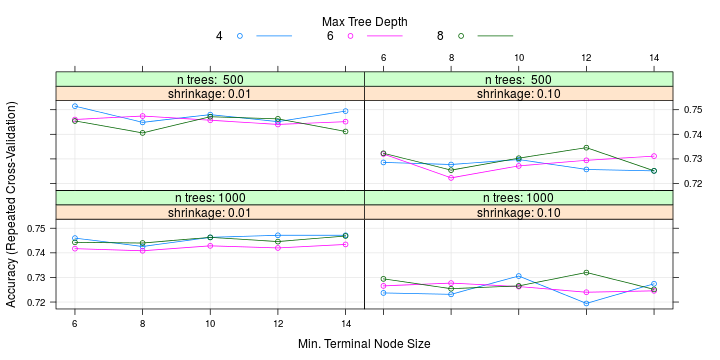Next, boosted trees on grouped variables:

``````set.seed(1)
mod_gbmG <- train(x = xTrainG,
y = yTrain,
method='gbm',
tuneGrid = gbmGrid,
verbose=F,
trControl = ctrl)

models\$gbmG <- mod_gbmG
``````

### C5.0

C5.0 is an example of a rule based tree.

``````set.seed(1)
c5_mod <- train(x = xTrain,
y = yTrain,
method     = "C5.0",
tuneLength = 10,
trControl  = ctrl)

models\$c5 <- c5_mod
plot(c5_mod)
``````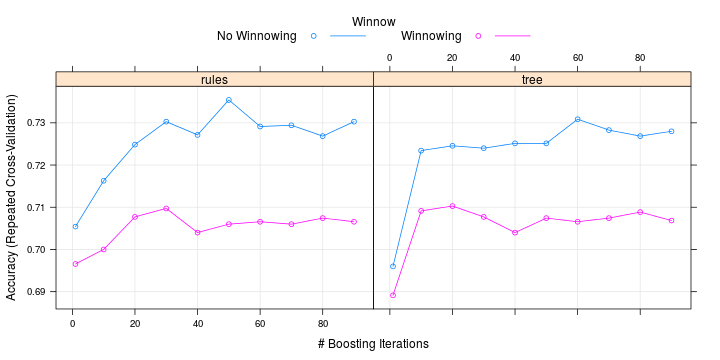``````set.seed(1)
c5_modG <- train(x = xTrainG,
y = yTrain,
method     = "C5.0",
tuneLength = 10,
trControl  = ctrl)

models\$c5G <- c5_modG
``````

## Results of model fitting

Let's present the results of model fitting and answer the following questions
that we formulated at the onset of this exercise:

• In-sample cross-validation:
• which models do better perform in-sample based on cross-validated results?
This is important for assessing generalization ability of the models.
• Out-of-sample validation:
• which models do better perform on held-out test sample? This is another,
more direct shot at generalization ability.
• Feature importance:
• what are the most important features? Do different models concur on what
are the most important features in advancing a credit?
• Feature interpretation:
• what are the positive/negative signs of creditworthiness?

### In-sample cross-validation

At this time we have `models` object that contains all our models. The models that are
objects of class `train`, among other things, held results of in-sample
cross-validation on held-out resamples.

Because resamples are the same for all models, which was ensured by:

1. common `ctrl` objects and
2. setting the same seed prior to resampling

we have the full right to assess the results of resampling via the following commands:

``````resamp <- resamples(models)
summary(resamp, metric='Accuracy')
``````
```##
## Call:
## summary.resamples(object = resamp, metric = "Accuracy")
##
## Models: rf, rfG, gbm, gbmG, c5, c5G
## Number of resamples: 50
##
## Accuracy
##        Min. 1st Qu. Median   Mean 3rd Qu.   Max. NA's
## rf   0.6714  0.7286 0.7571 0.7517  0.7714 0.8286    0
## rfG  0.6714  0.7286 0.7571 0.7577  0.7857 0.8714    0
## gbm  0.6571  0.7179 0.7500 0.7514  0.7821 0.8429    0
## gbmG 0.6429  0.7286 0.7571 0.7529  0.7857 0.8286    0
## c5   0.6000  0.7000 0.7429 0.7354  0.7679 0.8286    0
## c5G  0.6143  0.7143 0.7429 0.7471  0.7857 0.8429    0
```

Again, this is a meaningful procedure, because resamples are the same across all models.

NOTE:

we can even do a pairwise comparison of resampling results
via `summary(diff(resamp))`, which will assess if the models produce results that
are statistically different. But that is not of interest here.

We can visualize model performance as follows:

``````bwplot(resamp, metric='Accuracy')
``````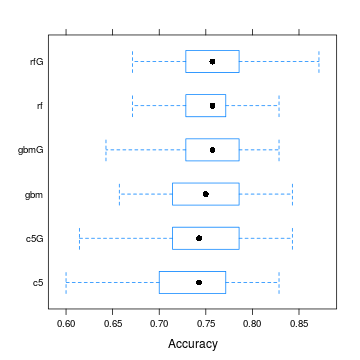### Out-of-sample validation

In-sample cross-validation is good for tuning hyperparameters. However, due to
cherry-picking, generalization ability measured by such cross-validation is
biased upward.

A fairer approach would be checking how our models perform on samples that they
never saw before, i.e. on held-out samples that were set aside at the beginning
of the exercise (see Split train/test section)

``````accuracy <- vector(mode='numeric')

for (i in names(models)) {
if (grepl('G\$', i)) {
accuracy[i] <- mean(predict(models[[i]], xTestG) == yTest)
} else {
accuracy[i] <- mean(predict(models[[i]], xTest ) == yTest)
}
}

accuracy
``````
```##        rf       rfG       gbm      gbmG        c5       c5G
## 0.7766667 0.7833333 0.7666667 0.7800000 0.7766667 0.7833333
```

We will visualize accuracies obtained:

``````dotchart(sort(accuracy), main="Out-of-sample model Accuracy")
``````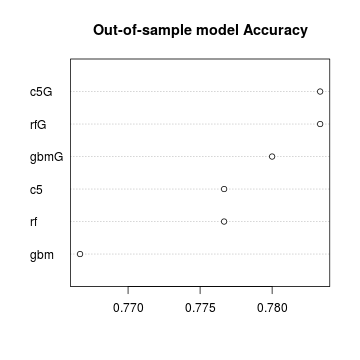### Feature importance

Another important step in interpreting the models is checking for consistency
among models for most important features.

To do so, let's extract feature importance with the help of caret's function
`varImp` and then plot top 10 features, side by side, with the help of
custom made `plotImp` funcion. This is a pretty wrapper for base R's `dotchart`
function.

``````par(mfrow=c(1,3))
rf_imp   <- varImp(models\$rf )
gbm_imp  <- varImp(models\$gbm)
c5_imp   <- varImp(models\$c5 , metric='splits')
plotImp(rf_imp,  main = '  RF  var importance', cex=1.1)
plotImp(gbm_imp, main = '  GBM var importance', cex=1.1)
plotImp(c5_imp,  main = ' C5.0 var importance', cex=1.1)
``````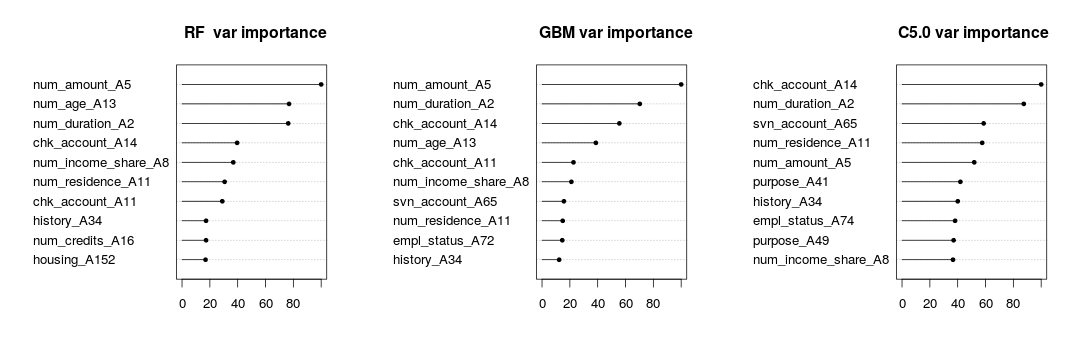### Feature interpretation

Even though the above plots show consistency among models for what are
considered to be the most important features, we still have no idea if big or small
`num_account_A5`, which is credit amount asked for, is a sign of positive
or negative credit decision. In other words, interpretability of the
ensemble black boxes is very low.

To overcome low interpretability let's build a regularized logistic model via
`glmnet` package that will be trained by caret's `train`

``````grid <- expand.grid(alpha = seq(0,.2,by=.01),
lambda = 10^(seq(-3,0,by=.5)))

set.seed(1)
glmnet_mod <- train(x = xTrain,
y = yTrain,
method = "glmnet",
tuneGrid = grid,
preProcess = c('center', 'scale'), # to ensure fair regularization
trControl = ctrl)

plot(glmnet_mod)
``````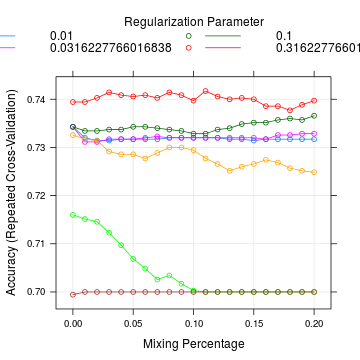To continue in parallel with our previous focus on feature importance,
let's define the features, that are left after normalization and regularization
with biggest coefficients, to be most important (i.e. outcome is most sensitive
to changes in these features).

NOTE:

as of now, `glmnet` function does not produce scores for statistical significance
of the coefficients, only regularized values.

Let's plot the so-defined most 'important' features:

``````coefGLM <- coef(glmnet_mod\$finalModel, glmnet_mod\$bestTune\$lambda)
glm_imp <- list()
glm_imp\$importance <- abs(coefGLM[order(abs(coefGLM), decreasing = T),])
plotImp(glm_imp, main = ' GLMNET var importance')
``````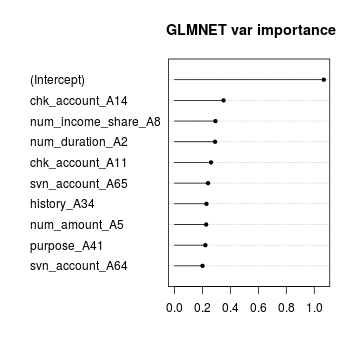Now we can see a significant overlap between non-linear tree ensemble methods
(RF, gbm, C5.0 above) and linear logistic regression, which is a very assuring sign!

Let's print out top 10 coefficients, to see the signs of the coefficients,
and ultimately to understand how most important features affect credit decision:

``````coefGLM[order(abs(coefGLM), decreasing = T),][1:10]
``````
```##         (Intercept)     chk_account_A14 num_income_share_A8
##          -1.0680333          -0.3513805           0.2931871
##     num_duration_A2     chk_account_A11     svn_account_A65
##           0.2904506           0.2617197          -0.2403409
##         history_A34       num_amount_A5         purpose_A41
##          -0.2291794           0.2270560          -0.2210416
##     svn_account_A64
##          -0.2008976
```

With this information we are ready to wrap up our analysis, to draw conclusions,
and to map our way forward in Python

## Summary and to-do list:

1. The most important features in deciding on client credit worthiness:

• amount of money asked for (`num_amount_A5`), `positive`
• age (`num_age_A13`), `positive`
• duration of credit (`num_duration_A2`), `positive`
• no availability of credit history (`chk_account_A14`), `negative`
• share of installment payments as of current income (`num_account_share_A8`), `positive`
• time spent in present residence (`num_residence_A11`), `positive`
• if the client has other credits (`chk_account_A11`), `positive`
• distressed accounts at other banks (`history_A34`), `negative`
• number of credit at this bank (`num_credits_A16`), `negative`
• ownership of housing (`housing_A152`), `positive`
2. Strange findings like:

• the higher the share of disposable income the higher the probability of
advancing the credit (positive `num_account_share_A8`, positive correlation to amount?)
• other credits at other banks (positive `history_A34`, others have checked this
person for you already?)

should be further investigated.

1. Other areas of investigation is probable non-linear relations of outcome to `age` (try quadratic?) and interaction between features, especially for `GLM` model.

2. `RF` and `gbm` appear to be winners in this competition with accuracies around 0.75, which
can be considered a benchmark for further model tuning.

3. Less complex models, like models with grouped variables and `GLM` appear to perform
better on this data set.

4. A finer model tuning is justified:

• for `gbm` and `RF`: bigger trees, wider range of tuning parameters
• for `GLM`: given small size of data set, more careful feature selection is worth trying, especially if interactions are introduced.
5. Model ensembling, e.g. `RF` + `GLM`, is worth trying and cross-validating.